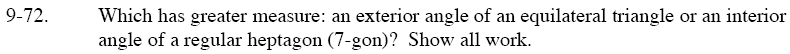### Home > GC > Chapter 9 > Lesson 9.2.2 > Problem9-72

9-72.Remember that the sum of all exterior angles is equal to 360°.

$\frac{360\degree}{3}=120\degree\text{ for each exterior angle, since it is an equilateral triangle.}$

$\text{Measure of one interior angle }=\frac{(n-2)(180)}{n}$

Substitute 7 for n and determine which of the two angles has a greater measure.